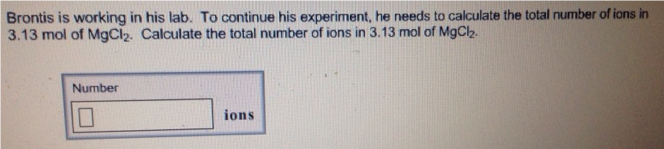# Problem: Brontis is working in his lab. To continue his experiment, he needs to calculate the total number of ions in 3.13 mol of MgCl2. Calculate the total number of ions in 3.13 mol of MgCl 2.

###### FREE Expert Solution
90% (366 ratings)###### Problem Details

Brontis is working in his lab. To continue his experiment, he needs to calculate the total number of ions in 3.13 mol of MgCl2. Calculate the total number of ions in 3.13 mol of MgCl 2